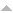431 sonucu aktar:
Yazar Başlık Tür [ Yıl]
2017
Güran, S.Z.., and H. Yaşarcan, Korean System Dynamics Review, vol. 17, pp. 81-110, 2017.
Mutallip, A.., and H. Yaşarcan, Korean System Dynamics Review, vol. 18, pp. 81-110, 2017.
Korean System Dynamics Review, vol. 4, pp. 81–110, Feb, 2017.
2018
Discrete Mathematics & Theoretical Computer Science, vol. 20, 2018.
Edali, M., and G. Yücel, Journal of Artificial Societies and Social Simulation, vol. 21, pp. 1, 2018.
Pattern Recognition, vol. 73, pp. 202 - 215, 2018.
Simulation and Modeling Methodologies, Technologies and Applications, Cham, Springer International Publishing, 2018.
Küçükaydın, H., and N. Aras, Sigma, vol. 9, pp. 371–382, 2018.
Başoğlu, İ., W. Hörmann, and H. Sak, Annals of Operations Research, vol. 260, pp. 113–128, 2018.
Discrete Mathematics, vol. 341, pp. 2859-2871, 2018.
Discrete Mathematics and Theoretical Computer Science, vol. 20, pp. 1b–1b, 2018.
Metin, E., and G. Kumbaroğlu, International Journal of Energy Economics and Policy, vol. 8, pp. 141–149, 2018.
Ahat, B., T. Ekim, and Z. C. Taşkın, INFORMS Journal on Computing, vol. 30, pp. 43-56, 2018.
Ekim, T., A. Boyacı, and M. Shalom, Journal of Combinatorial Optimization, vol. 35, pp. 250–265, Jan, 2018.
Survey Methodology, vol. 44, pp. 1–16, Jun, 2018.
International Journal of Production Economics, vol. 197, pp. 13-26, 2018.
International Journal of Production Research, vol. 56, pp. 3344-3357, 2018.
Barlas, Y., and O. Özgün, Journal of Simulation, vol. 12, pp. 144-161, 2018.
Aras, N., and Ü. Bilge, Applied Mathematical Modelling, vol. 60, pp. 526-539, 2018.
Journal of Economics and Political Economy, vol. 5, pp. 234–249, Jun, 2018.
2019
Computers & Operations Research, vol. 111, pp. 214-229, 2019.
Annals of Operations Research, vol. 279, pp. 1–42, 2019.
Turkish Journal of Electrical Engineering & Computer Sciences, vol. 27, pp. 832–846, 2019.
Physics in Medicine {&} Biology, vol. 64, pp. 205024, oct, 2019.
Şeker, O., T. Ekim, and Z. C. Taşkın, Networks, vol. 73, pp. 145-169, 2019.
European Journal of Operational Research, vol. 272, pp. 372-388, 2019.
Deniz, Z., and T. Ekim, Discrete Applied Mathematics, vol. 261, pp. 136-147, 2019.
Gürel, Y., and R. Güllü, International Journal of Production Economics, vol. 209, pp. 112-120, 2019.
IEEE Transactions on Intelligent Transportation Systems, vol. 20, pp. 4635-4642, 2019.
Edali, M., and G. Yücel, Simulation Modelling Practice and Theory, vol. 92, pp. 62-81, 2019.